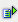The function len (string) returns the number of characters of a text string.

Syntax: =len(string)Example Cell A1 = abc =len(A1) is 3 Cell A1 = abc =len(A1) is 8